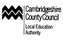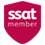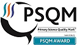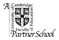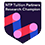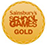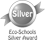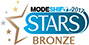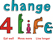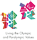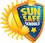# Learning expectations in Year 3

By the end of Year 3 children should be able to...

• I can apply knowledge of root words, prefixes and suffixes to read aloud and to understand the meaning of unfamiliar words.
• I can read further exception words, noting the unusual correspondences between spelling and sound.
• I attempt pronunciation of unfamiliar words drawing on prior knowledge of similar looking words.

• I read a range of fiction, poetry, plays, and non-fiction texts.
• I can discuss the texts that I read.
• I can read aloud and independently, taking turns and listening to others.
• I can explain how non-fiction books are structured in different ways and can use them effectively.
• I can explain some of the different types of fiction books.
• I can ask relevant questions to get a better understanding of a text.
• I can predict what might happen based on details I have.
• I can draw inferences such as inferring a characters’ feelings, thoughts and motives from their actions.
• I can use a dictionary to check the meaning of unfamiliar words.
• I can identify the main point of a text.
• I can explain how structure and presentation contribute to the meaning of texts.
• I can use non-fiction texts to retrieve information.
• I can prepare poems to read aloud and to perform, showing understanding through intonation, tone, volume and action.

Spelling

• I can spell words with additional prefixes and suffixes and understand how to add them to root words.
• I recognise and spell homophones.
• I can use the first two or three letters of a word to check its spelling in a dictionary.
• I can spell words correctly which are in a family.
• I can spell the commonly mis-spelt words from the Y3/4 word list.
• I can identify the root in longer words.

Handwriting

• I use the diagonal and horizontal strokes that are needed to join letters.
• I understand which letters should be left unjoined

Sentence structure

• I can express time, place and cause by using conjunctions, adverbs and prepositions.

Text structure

• I am starting to use paragraphs.
• I can use the present perfect form of verbs instead of the simple past.

Punctuation

• I use capital letters for names of people, places, day of the week and the personal pronoun ‘I’.
• I correctly use question marks and exclamation marks,
• I can use commas to separate items in a list.
• I can use apostrophes to show where letters are missing and to mark singular possession in nouns.
• I can use inverted commas to punctuate direct speech.

Number
• I can compare and order numbers to 1000 and read and write numbers to 1000 in numerals and words.
• I can count from 0 in multiples of 4, 8, 50 and 100.
• I can recognise the value of each digit in a 3-digit number.
• I understand and can count in tenths, and find the fractional value of a given set.
• I can add and subtract fractions with a common denominator.
• I can derive and recall multiplication facts for 3, 4 and 8x tables.
• I can add and subtract mentally combinations of 1-digit and 2- digit numbers.
• I can add and subtract numbers with up to 3-digits using formal written methods.
• I can write and calculate mathematical statements for multiplication and vision using the 2x, 3x, 4x, 5x, 8x and 10x tables.
• I can calculate 2-digit x 1-digit.
• I can solve number problems using one and two step problems

Measurement, geometry and statistics

• I can identify right angles and can compare other angles stating whether they are greater or smaller than a right angle.
• I can identify horizontal and vertical lines and pairs of perpendicular and parallel lines.
• I can tell the time to the nearest minute and use specific vocabulary, including seconds, am & pm.
• I can measure, compare, add and subtract using common metric measures.
• I can solve one and two step problems using information presented in scaled bar charts, pictograms and tables.